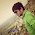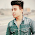# How to find Time Complexity of an Algorithm

We can come up with several algorithms for a particular problem. But we need to choose the best algorithm that suits us. Hence we need to compare several algorithms and select the best algorithm.

Therefore, goal of analysis of algorithms is to compare algorithms with several factors like running time, memory, effort of developing, etc. Ideal factor to be selected for comparison purpose is running time of the algorithm which is a function of input size, n. Since running time is a function of input size it is independent of execution time of the machine, style of programming etc.
Below are some examples with the help of which you can determine the time complexity of a particular program (or algorithm).

```main(){
int a=10,b=20,sum; //constant time, say c1
sum = a + b; //constant time, say c2
}```
The time complexity of the above program = O(1)

How did we get O(1). First calculate the total time of each statement in the program (or algorithm). I have commented the time taken for each line. Why constant time? Because the statement to be executed does not depend on the input size. So it takes some constant execution time which depends on the machine. Since it does not depend on the input size we consider it as a constant time say c. So the total time taken by the program is
Total Time =  c1 + c
Therefore Time Complexity = O(1)

What does Big-O Notation mean?
This notation gives the upper bound of a given function. for example, if
f(n) = n3 + 25n2 + n + 10
the upper bound of f(n) is n3. That means n3 gives the maximum rate of growth for f(n) at larger values of n. Therefore f(n) = O(n3)

Lets us return back to determine the time complexity.

See another example:
```for(i=1; i<=n; i++)
sum = sum + 2; // constant time, c
```
The above code is a for loop. We know that the body of the for loop will be executed n times. Since the body of the loop consists of only one statement and since it takes only constant time c to execute the total time taken by the for loop is, Total time = nc

Here f(n) = nc, therefore Time Complexity = O(n)

Another example:
```for(i=1; i<=n; i++)
i = i * 2; // constant time, c```
Here we can see that the value of i is doubling on every iteration. i.e At first iteration i = 2, second iteration i = 4, third iteration i = 8. Assume that at kth iteration, value of i reaches n. That is, 2k = n

Taking log on both sides we get
log(2k) = log n
k log2 = log n
k = log n
Therefore time complexity = O(log n)

Another example:
```for(i=1; i<=n; i++){
for(j=1; i<=n; i++)
sum = sum + 2; // constant time, c
}```
In the above code we can see nested loops. The inner loop iterates n times and outer loop iterates n time. Therefore, total time = c n n = cn2 = O(n2)

Another example:
```sum = a + b; //constant time, say c
for(i=1; i<=n; i++)
i = i * 2; // constant time, d
for(i=1; i<=n; i++){
for(j=1; i<=n; i++)
sum = sum + 2; // constant time, e
}```
Total time = c + dn + en2 = O(n2)

Another example:
```if(i==1) //constant time for executing condition,c
return false; //constant time, d
else{
for(j=1; i<=n; i++)
sum = sum + 2; // constant time, e
}```
Total time = c + d + e n = O(n)

Another example:
```if(i==1) //constant time for executing condition,c
return false; //constant time, d
else
for(j=1; i<=n; i++){
if(i>1) //constant time,f
sum = sum + 2; //constant time, e
}```
Total time = c + d + ( f + e  ) n = O(n)

Another example:
```if(i==1){ //constant time for executing condition,c
for(i=1; i<=n; i++)
for(j=1; i<=n; i++)
sum = sum + 2; // constant time, d
}else{
for(j=1; i<=n; i++)
sum = sum + 2; // constant time, e
}```
Total time = c + dn2 + e n = O(n2)

1.how to calculate complexity of a program using C program?
I know how to calculate it theoritically, but unable to implement it in C program itself. please suggest some method. THANK YOU!

1.I think the author has given some examples with which you can try to find the complexity of your program.

2.i have question can you give me solution then plz..

for(int i=0;i<n;i++)
{
for(int j=0;j<=i;j++)
{
sum++;
}
}

what is time complexity of this program?

3.O(n2) because

for(int i=0;i<n;i++)
{
for(int j=0;j<=i;j++)
{
sum++; //constant time c
}
}

inner loop depends on the input n as its limit till 0 to n. and for time complexity we have to choose upper limit that is O(c*0+c*1+c*2.....+c*n)=O(n) and for whole program=O(n2).

4.func(inta[], int n){for(i=1;i<=n;i++) sum=sum+a[i];} find time complexity

5.what is the complexity for following program

for(i=0; i<n;)
i=i*k;

assuming k to be a constant.

1.6.Hii...please find the time complexity of this algorithm..

1) Sort the received data set, X , in ascending order.
2) Divide the set X into two halves, lower half, X[LH]
and upper half, X[UH]. Let X[LH] =(L1 < L2 < L3 ...... Lh) and X[UH] =(U1
< U2 < U3 ...... Uh)
3) For each half, X[LH] and X[UH] , calculate the gaps
between successive data points.
4) Find the position of the largest gap, Pos[LH] , from the
set X[LH] for finding the lower outlier.
5) Similarly, find the position of the largest gap, Pos[UH],
from the set X[UH] for finding the upper outlier.
6) The number of data points to the left of the Pos[LH]
are the detected lower outlier and the number of
data points to the right of the Pos[UH], are the detected
upper outliers

7.8.i have a question can u solve
1. Calculate the Time complexity of the Algorithm below:
A()
{
int I,j,k;
for(i=n/2;i<=n;i++)
for(j=1;j<=n;j=2*j)
for(k=1;k<=n;k=k*2) printf(“KLEF”);
}

9.{
int I,j,k;
for(i=n/2;i<=n;i++)
for(j=1;j<=n;j=2*j)
for(k=1;k<=n;k=k*2) printf(“KLEF”);
}

10.,,,,,,,,,,,,,,,,,,,

11.Find the complexity of the below program:
function(int n)
{
if (n==1)
return;
for (int i=1; i<=n; i++)
{
for (int j=1; j<=n; j++)
{
printf("*");
break;
}
}

12.ans for the above question